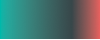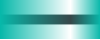1. A linear gradient should be given a `id` attribute so that it can be used by other elements as a fill. Make sure that the value contains only letters and numbers and is unique in your SVG.

2. The gradient can be defined horizontally or vertically. You specify this as a percentage or in absolute values.

Horizontally:

```<linearGradient x1="0" y1="0" x2="100" y2="0">
```

Vertically:

```<linearGradient x1="0" y1="0" x2="0" y2="100">
```
3. Each color step within a gradient is defined with an `<stop>` element that determines the color value and transparency.

The `offset` attribute specifies where the color change is to take place. Use values between 0 and 1 for this. If you do not define any offsets, the color changes will automatically be evenly distributed over the entire gradient.

4. The attribute `stop-color` defines the desired color value. Various notations are permitted:

```stop-color="#F08080"
```
```stop-color="lightcoral"
```
```stop-color="rgb(240,128,128)"
```
5. The attribute `stop-opacity` defines the transparency or opacity. Use values between 0 and 1 for this, where 0 is completely transparent and 1 is completely opaque. If no value is explicitly specified, 1 is used.

## Example with transparency

```<linearGradient id="myGradient" x1="0%" y1="0%" x2="100%" y2="0%">
<stop offset="0.0" stop-color="#00B8AA" />
<stop offset="0.7" stop-color="#00B8AA" stop-opacity="0" />
<stop offset="1.0" stop-color="#00B8AA" />
```<rect x="0" y="15" fill="#37464a" width="100" height="10" />